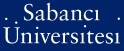# On invariants of towers of function fields over finite fields

Hess, Florian and Stichtenoth, Henning and Tutdere, Seher (2013) On invariants of towers of function fields over finite fields. Journal of Algebra and Its Applications, 12 (4). ISSN 0219-4988 (Print) 1793-6829 (Online)

This is the latest version of this item.

Full text not available from this repository.

Official URL: http://dx.doi.org/10.1142/S0219498812501903

## Abstract

In this paper, we consider a tower of function fields F = (F-n)(n >= 0) over a finite field F-q and a finite extension E/F-0 such that the sequence epsilon := E . F = (EFn)(n >= 0) is a tower over the field F-q. Then we study invariants of epsilon, that is, the asymptotic number of the places of degree r in epsilon, for any r >= 1, if those of F are known. We first give a method for constructing towers of function fields over any finite field F-q with finitely many prescribed invariants being positive. For q a square, we prove that with the same method one can also construct towers with at least one positive invariant and certain prescribed invariants being zero. Our method is based on explicit extensions. Moreover, we show the existence of towers over a finite field F-q attaining the Drinfeld-Vladut bound of order r, for any r >= 1 with q(r) a square (see [1, Problem-2]). Finally, we give some examples of non-optimal recursive towers with all but one invariants equal to zero.

Item Type: Article Article Number: 1250190 Towers of function fields; genus; number of places Q Science > QA Mathematics 21697 Henning Stichtenoth 23 Jul 2013 11:40 17 Sep 2015 14:29

### Available Versions of this Item

Repository Staff Only: item control page